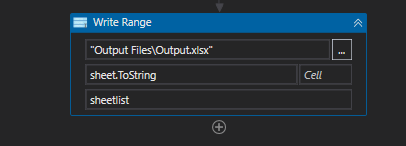# How to copy this column header into new excel

I need some help in my excel i want to be dyanmic read since my sheet now is dynamic where i wouldnt know the name as this will change everytime i wan to find in excel this column name or row name call Cost Price or Project ID then copy the value below it into a new excel output but i meet a difficulty where in my 2nd sheet this cost centre is not join together
how am i able to do it ? Any help is approciated

The instruction is like this
1)Use Excel application Scope
2)Get Workbook sheet
3)For each loop through each sheet on the workbook sheet
4)For each loop loop through column a and b and loot through first row to last non empty row to look for Cost Price if not found move on the next sheet
5)start from cell contain Cost Price check for next column to see if is Project ID

I have attached an example test price excel
Test Price.xlsx (10.4 KB)

Hello @xxGoRpa

1. Use Excel Application Scope:

• WorkbookPath: “your_file_path.xlsx”
2. For Each Sheet in Workbook:

• For each sheet in the workbook, do the following:
1. For Each Column in A to Z:

• For each column from A to Z, do the following:
1. Read Cell for “Cost Price”:

• Assign activity:
• Left: cellValue1 (String)
• Right: Excel Read Cell activity
• Workbook: workbook variable
• SheetName: sheet variable
• Cell: “A” + startRow variable
2. While cellValue1 is not empty:

• Use a While activity to iterate while cellValue1 is not empty.
1. Read Cell for “Project ID”:

• Assign activity:
• Left: cellValue2 (String)
• Right: Excel Read Cell activity
• Workbook: workbook variable
• SheetName: sheet variable
• Cell: “B” + startRow variable
2. Output the values or perform desired actions:

• Use the extracted cell values (cellValue1 and cellValue2) for further processing or output.
3. Increment startRow:

• Assign activity:
• Left: startRow variable
• Right: startRow + 1

Thanks & Cheers!!!

Hi currently i cant use the read cell activity as currently this is unknow i only can literate each row till i find the Cost Project

Hi @xxGoRpa ,
you want to find index in sheet 2nd, that’s right
We can get sheet and index of columns by index too
alphabet can convert o number…
we can read all then use for each then find index of it
regards,

can give example of the xaml so i can take a look like how to do
my final output is it write range to an new excel with the sheet name but it will display the Cost Price and Project ID if there is one

we need find and get the Cost Price and Project ID in 1st sheet

then we need find and get the Cost Price and Project ID in 2nd sheet

write value of the Cost Price and Project ID in 1st sheet to new index in 2nd
that’s right?

correct Cost Price is definitely there but Project ID is optional it may be there or not !

Hi @xxGoRpa

Excel Application Scope (WorkbookPath: “YourExcelFilePath.xlsx”)
Get Workbook Sheets → Output: sheetNames

``````For Each sheet In sheetNames
Read Range from sheet → Output: dtCurrentSheet

For Each row In dtCurrentSheet.Rows
If row("A").ToString.Contains("Cost Price") OrElse row("B").ToString.Contains("Project ID") Then
' Perform actions to store/copy the values you need
' Use 'dtCurrentSheet.Rows.IndexOf(row) + 1' to get the next row index
' Retrieve values using dtCurrentSheet.Rows(nextRowIndex)("ColumnNameOrIndex").ToString
' Store these values in variables or a collection

' Exit the loop if necessary, using 'Exit For'
End If
Next row

' Use conditional logic here to check if you have found the values
' If found, exit the outer loop to stop processing further sheets
Next sheet
``````

’ Outside of the loops
’ Write the stored values to a new Excel file using Write Range or Write Cell

@xxGoRpa

1. Use Excel Application Scope with your file path.

2. For Each Sheet in the workbook:

• For each sheet, iterate through the rows.
3. Inside the row loop:

• Read the cell in the current row and first column (Assuming the “Cost Price” header is in the first column).
• If the cell contains “Cost Price”:
• Read the “Project ID” cell in the current row and second column.
• Process or output the values.
• Move to the next row.

I kind of stuck here as firstly Cost price in sheet 1 and sheet 2 they are different
which will result in an error if i use this

and 2nd thing how do we copy the column from cost price and project id ?However i copy all the column in my output why ??